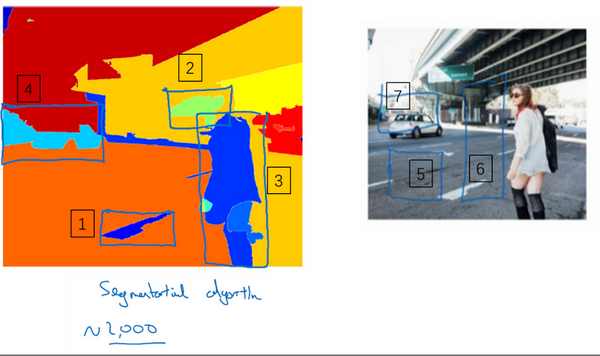## 第三周 目标检测（Object detection）

### 3.1 目标定位（Object localization）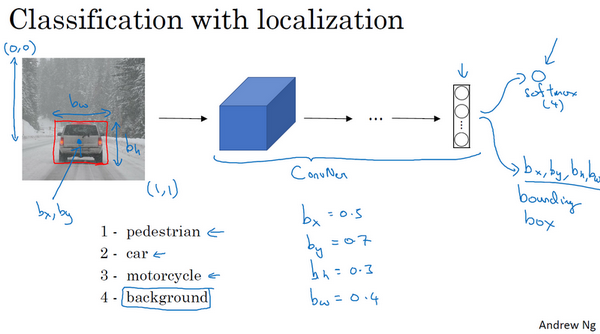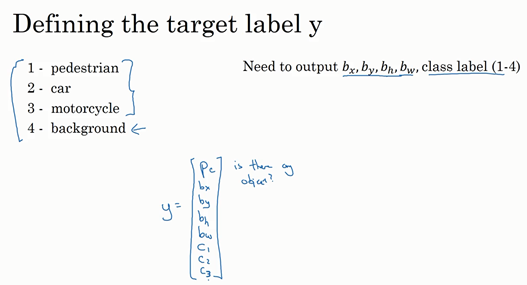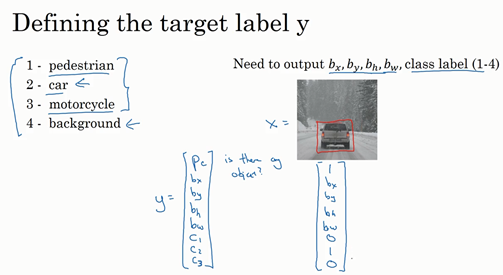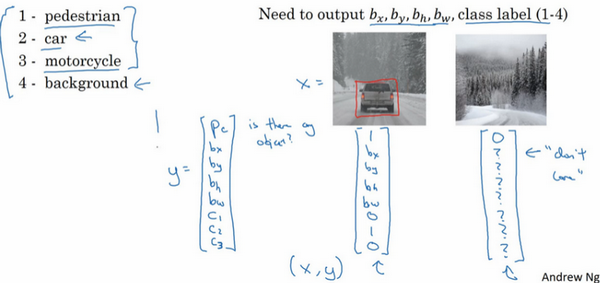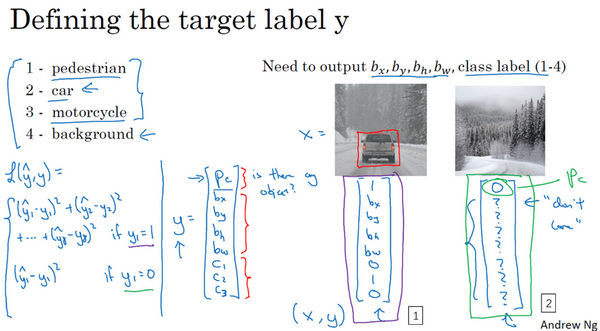### 3.2 特征点检测（Landmark detection）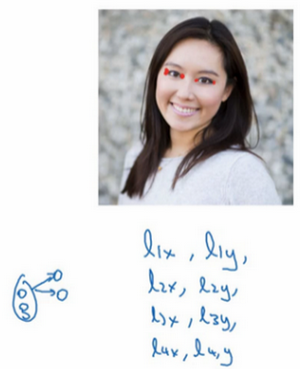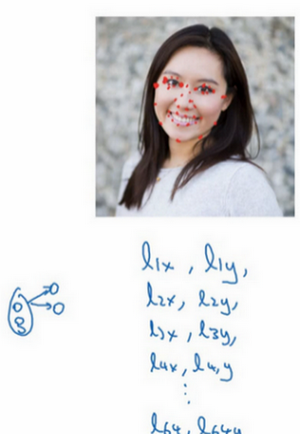### 3.3 目标检测（Object detection）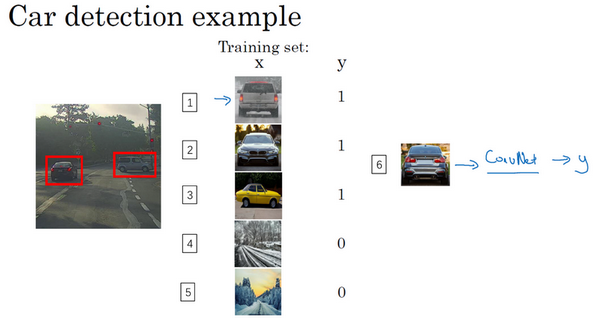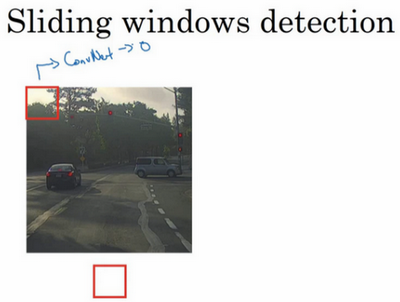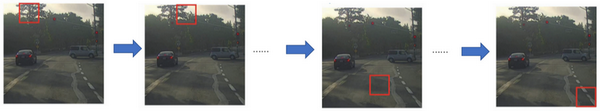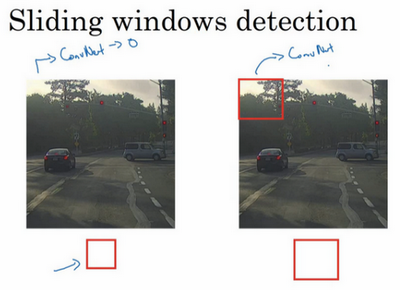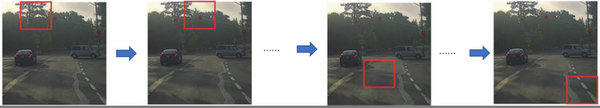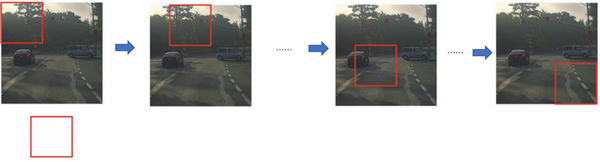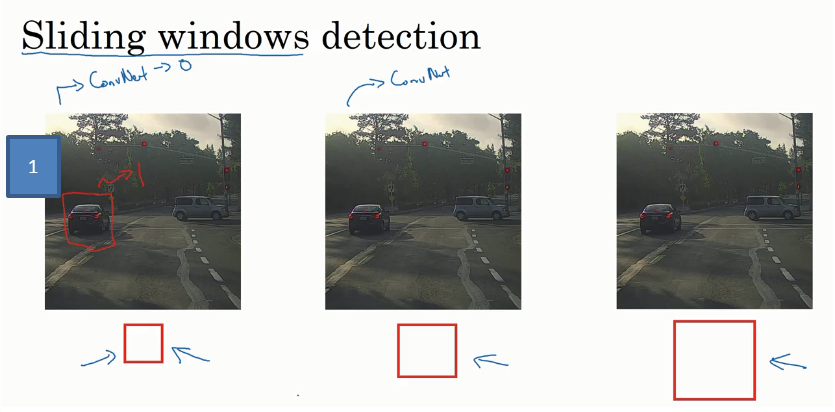### 3.4 滑动窗口的卷积实现（Convolutional implementation of sliding windows）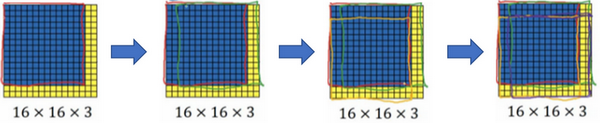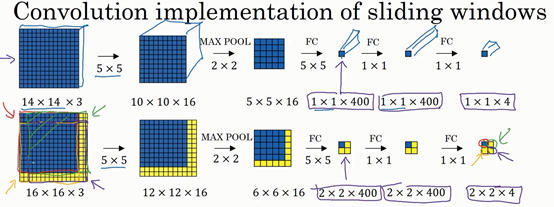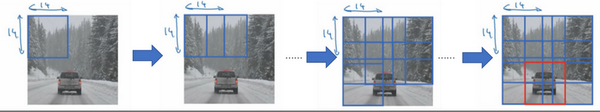### 3.5 Bounding Box预测（Bounding box predictions）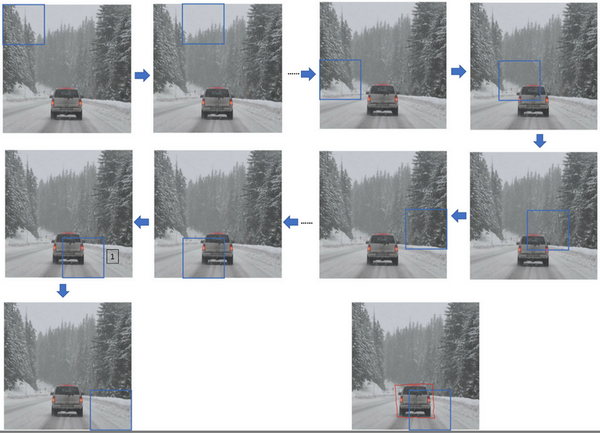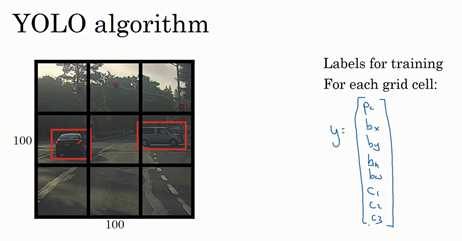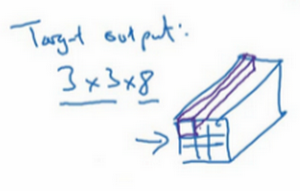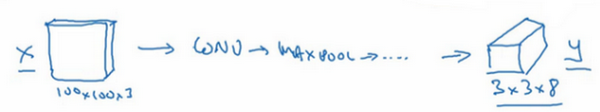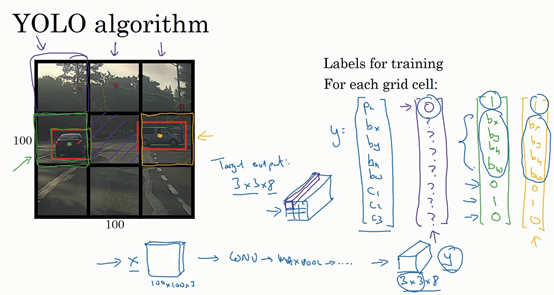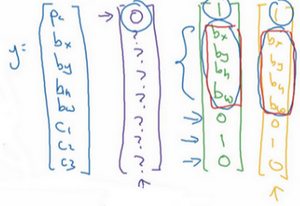Specify the bounding boxesYOLO算法中，对于这个方框（编号1所示），我们约定左上这个点是$(0,0)$，然后右下这个点是$(1,1)$,要指定橙色中点的位置，$b_{x}$大概是0.4，因为它的位置大概是水平长度的0.4，然后$b_{y}$大概是0.3，然后边界框的高度用格子总体宽度的比例表示，所以这个红框的宽度可能是蓝线（编号2所示的蓝线）的90%，所以$b_{h}$是0.9，它的高度也许是格子总体高度的一半，这样的话$b_{w}$就是0.5。换句话说，$b_{x}$$b_{y}$$b_{h}$$b_{w}$单位是相对于格子尺寸的比例，所以$b_{x}$$b_{y}$必须在0和1之间，因为从定义上看，橙色点位于对象分配到格子的范围内，如果它不在0和1之间，如果它在方块外，那么这个对象就应该分配到另一个格子上。这个值（$b_{h}$$b_{w}$）可能会大于1，特别是如果有一辆汽车的边界框是这样的（编号3所示），那么边界框的宽度和高度有可能大于1。

Redmon, Joseph, et al. "You Only Look Once: Unified, Real-Time Object Detection." (2015):779-788.

### 3.6 交并比（Intersection over union）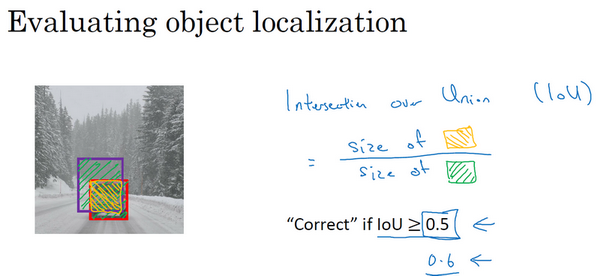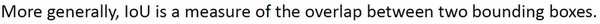### 3.7 非极大值抑制（Non-max suppression）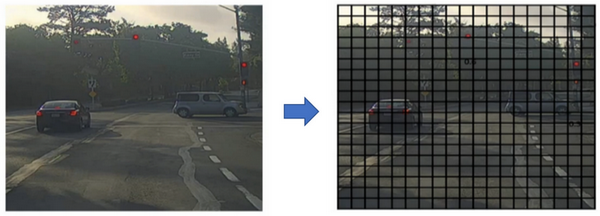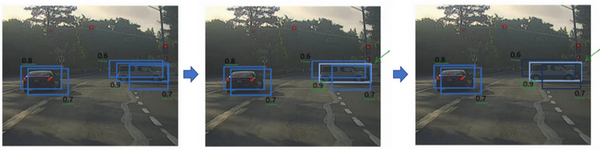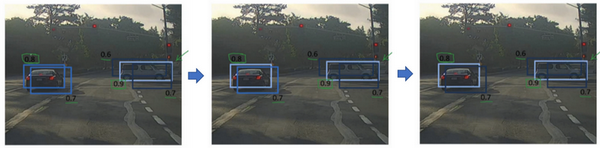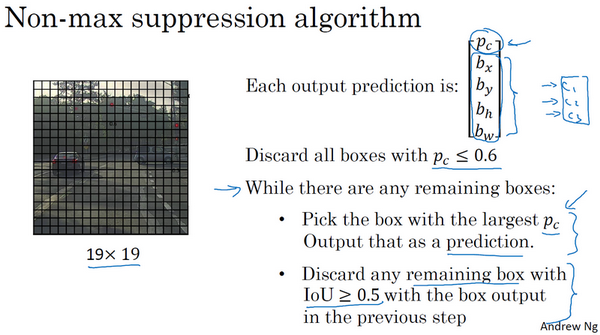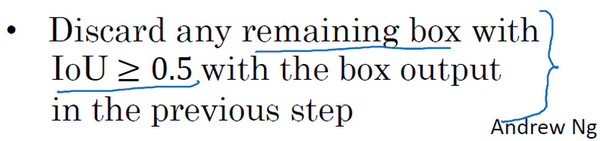### 3.8 Anchor Boxes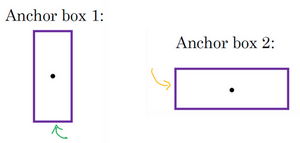anchor box的思路是，这样子，预先定义两个不同形状的anchor box，或者anchor box形状，你要做的是把预测结果和这两个anchor box关联起来。一般来说，你可能会用更多的anchor box，可能要5个甚至更多，但对于这个视频，我们就用两个anchor box，这样介绍起来简单一些。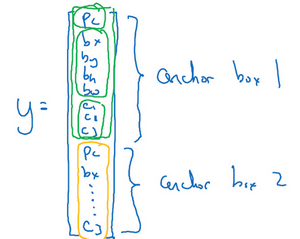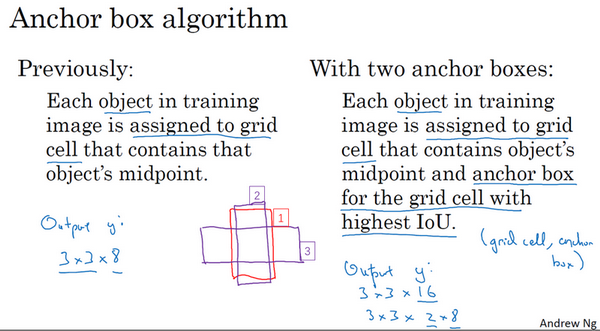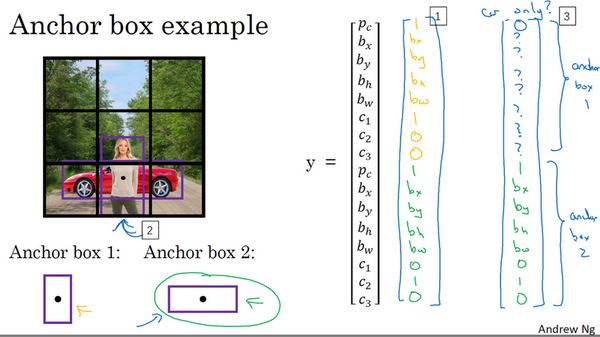$y =\begin{bmatrix} p_{c} & b_{x} & b_{y} & b_{h} & b_{w} & c_{1} & c_{2} & c_{3} &p_{c} & b_{x} & b_{y} & b_{h} & b_{w} & c_{1} & c_{2} & c_{3} \\\end{bmatrix}^{T}$

### 3.9 YOLO 算法（Putting it together: YOLO algorithm）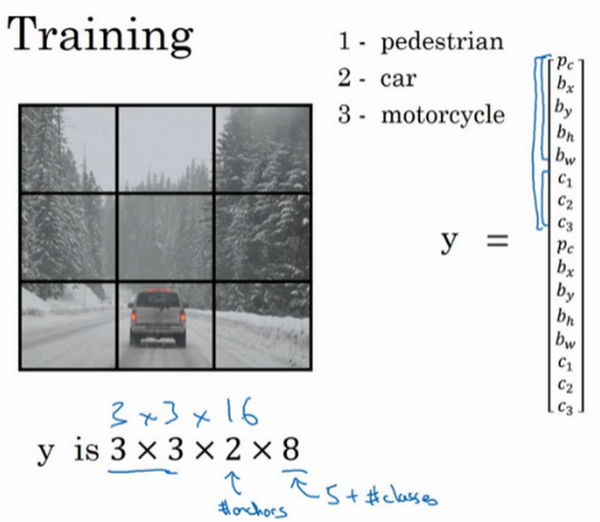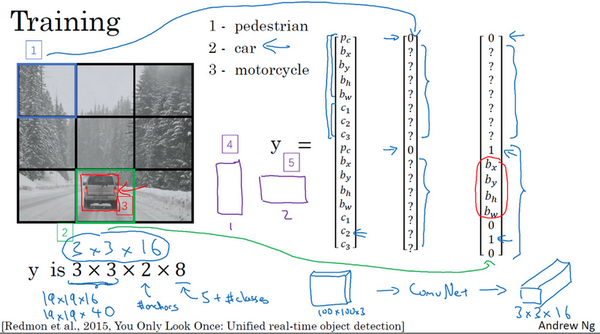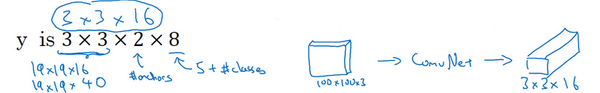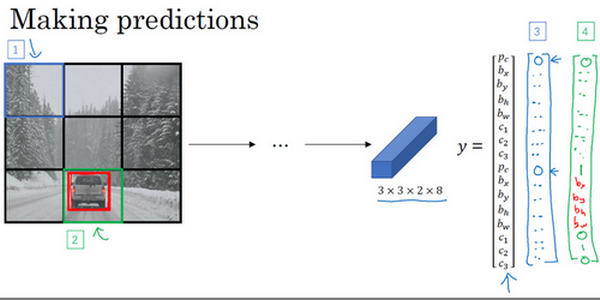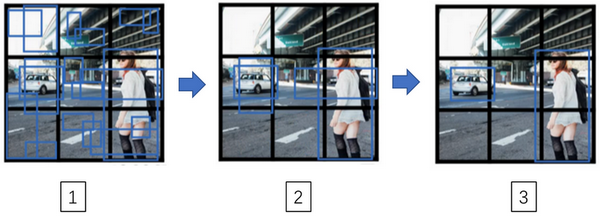### 3.10 候选区域（选修）（Region proposals (Optional)）[Girshick R, Donahue J, Darrell T, et al. Rich feature hierarchies for accurate object detection and semantic segmentation[C]//Proceedings of the IEEE conference on computer vision and pattern recognition. 2014: 580-587.]